International
Tables for
Crystallography
Volume B
Reciprocal space
Edited by U. Shmueli

International Tables for Crystallography (2006). Vol. B, ch. 5.1, pp. 537-538   | 1 | 2 |

## Section 5.1.2.6. Propagation direction

A. Authiera*

aLaboratoire de Minéralogie-Cristallographie, Université P. et M. Curie, 4 Place Jussieu, F-75252 Paris CEDEX 05, France
Correspondence e-mail: authier@lmcp.jussieu.fr

#### 5.1.2.6. Propagation direction

| top | pdf |

The energy of all the waves in a given wavefield propagates in a common direction, which is obtained by calculating either the group velocity or the Poynting vector [see Section A5.1.1.4, equation (A5.1.1.8)of the Appendix]. It can be shown that, averaged over time and the unit cell, the Poynting vector of a wavefield is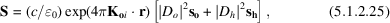where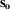and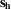are unit vectors in the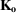and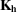directions, respectively, c is the velocity of light and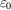is the dielectric permittivity of a vacuum. This result was first shown by von Laue (1952)in the two-beam case and was generalized to the n-beam case by Kato (1958).

From (5.1.2.25)and equation (5.1.2.22)of the dispersion surface, it can be shown that the propagation direction of the wavefield lies along the normal to the dispersion surface at the tie point (Fig. 5.1.2.5). This result is also obtained by considering the group velocity of the wavefield (Ewald, 1958; Wagner, 1959). The angle α between the propagation direction and the lattice planes is given by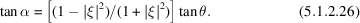It should be noted that the propagation direction varies betweenandfor both branches of the dispersion surface.

### References

Ewald, P. P. (1958). Group velocity and phase velocity in X-ray crystal optics. Acta Cryst. 11, 888–891.Google Scholar
Kato, N. (1958). The flow of X-rays and material waves in an ideally perfect single crystal. Acta Cryst. 11, 885–887.Google Scholar
Laue, M. von (1952). Die Energie Strömung bei Röntgenstrahl interferenzen Kristallen. Acta Cryst. 5, 619–625.Google Scholar
Wagner, E. H. (1959). Group velocity and energy (or particle) flow density of waves in a periodic medium. Acta Cryst. 12, 345–346.Google Scholar